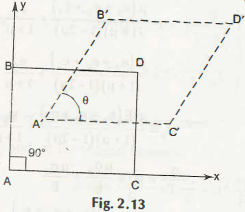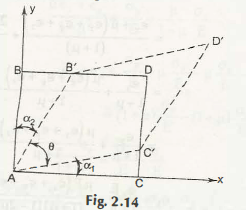If the faces AB and AC are two adjacent faces and perpendicular to each other, and along with that they are also perpendicular to the x axis and y axis. Just after deformation the AB sides take place of A’B’ and AC side takes place of A’C’. Now, the angle formed by B’A’C’ is not perpendicular or 90˚.  It means shear strain can be defined as the angle less than 90 degree. Thus, Yxy = 90° – θ. This is the angle subtracted from 90 degree and this is the value of shear strain.Now, if you consider this in a different way, suppose the displacement takes place from B to B’ in the x direction and C to C’ in y direction, then

The value of shear strain can be provided as Yxy =     900 – θ

= (a1 + a2)Now, you can easily distinguish between the different conditions to find out the shear Strain.

Links of Next Mechanical Engineering Topics:-### Customer Reviews

My Homework Help
Rated 5.0 out of 5 based on 510 customer reviews at